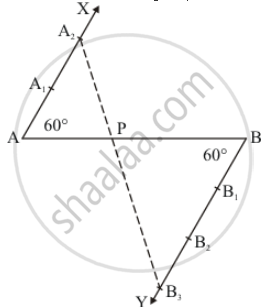Share

# Draw a Line Segment of Length 7 Cm and Divide It Internally in the Ratio 2 : 3. - CBSE Class 10 - Mathematics

#### Question

Draw a line segment of length 7 cm and divide it internally in the ratio 2 : 3.

#### Solution

Given that

Determine a point which divides a line segment of length 7 cm internally in the ratio of 2 : 3.

We follow the following steps of constructionStep of construction

Step: I- First of all we draw a line segment AB = 7 cm

Step: II- We draw a ray AX making an acute angle angleBAX = 60^@ with AB.

Step: III- Draw a ray BY parallel to AX by making an acute angle angleABY = angleBAX

Step IV - Mark of two points A_1, A_2 on AX and three points B_1, B_2,B_3on BY in such a way that "AA"_1 = A_1A_2 = BB_1 = B_1B_2 = B_2B_3

Step V - Join A_2B_3 and this line intersects AB at a point P

Thus P is the point dividing AB internally in the ration of 2:3

Is there an error in this question or solution?

#### APPEARS IN

Solution Draw a Line Segment of Length 7 Cm and Divide It Internally in the Ratio 2 : 3. Concept: Division of a Line Segment.
S